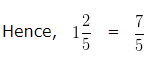# How to convert a Mixed Fraction into an Improper Fraction

To Convert Mixed Fraction to Improper Fraction, we follow the steps given below:-

Step 1: Multiply the Whole Number Part and Denominator of the Mixed Fraction

Step 2: Add the Numerator to the product obtained in Step 1

Step 3: Write the sum as the numerator and denominator would remain the same, as in the Mixed Fraction.

## How to convert a Mixed Fraction into an Improper Fraction?

Question 1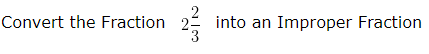Explanation

A combination of Whole Number and a Proper Fraction is called a Mixed Fraction

Here,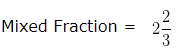Whole Number Part = 2

Numerator = 2

Denominator = 3

Step 1: Multiply the Whole Number Part and Denominator of the Mixed Fraction

2 x 3 = 6

Step 2: Add the Numerator to the product obtained in Step 1

2 + 6 = 8

Write the sum as the numerator and denominator would remain the same, as in the Mixed Fraction.
8/3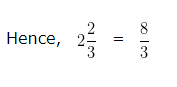Question 2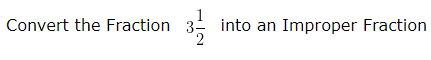Explanation

A combination of Whole Number and a Proper Fraction is called a Mixed Fraction

Here,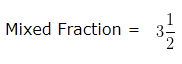Whole Number Part = 3

Numerator = 1

Denominator = 2

Step 1: Multiply the Whole Number Part and Denominator of the Mixed Fraction

3 x 2 = 6

Step 2: Add the Numerator to the product obtained in Step 1

1 + 6 = 7

Write the sum as the numerator and denominator would remain the same, as in the Mixed Fraction.

7/2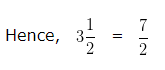Question 3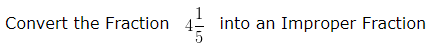Explanation

A combination of Whole Number and a Proper Fraction is called a Mixed Fraction

Here,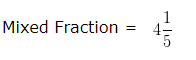Whole Number Part = 4

Numerator = 1

Denominator = 5

Step 1 : Multiply the Whole Number Part and Denominator of the Mixed Fraction

4 x 5 = 20

Step 2 : Add the Numerator to the product obtained in Step 1

1 + 20 = 21

Write the sum as the numerator and denominator would remain the same , as in the Mixed Fraction.

21/5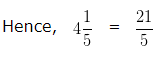Question 4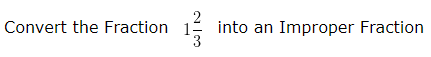Explanation

A combination of Whole Number and a Proper Fraction is called a Mixed Fraction

Here,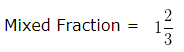Whole Number Part = 1

Numerator = 2

Denominator = 3

Step 1 : Multiply the Whole Number Part and Denominator of the Mixed Fraction

1 x 3 = 3

Step 2 : Add the Numerator to the product obtained in Step 1

2 + 3 = 5

Write the sum as the numerator and denominator would remain the same , as in the Mixed Fraction.

5/3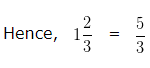Question 5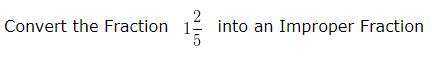Explanation

A combination of Whole Number and a Proper Fraction is called a Mixed Fraction

Here,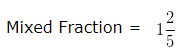Whole Number Part = 1

Numerator = 2

Denominator = 5

Step 1 : Multiply the Whole Number Part and Denominator of the Mixed Fraction

1 x 5 = 5

Step 2 : Add the Numerator to the product obtained in Step 1

2 + 5 = 7

Write the sum as the numerator and denominator would remain the same , as in the Mixed Fraction.

7/5